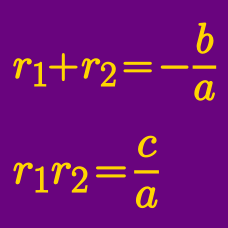Algebra

# Vieta's Formula Problem Solving

Suppose one of the roots of the quadratic equation $x^2+6ax+b=0$ is $\sqrt{3+\sqrt{8}}.$ If $a$ and $b$ are rational numbers, what is the value of $15ab?$

What is the sum of the two roots of the following equation: $(3x-1355)^2+4(3x-1355)+5=0?$

$A$ and $B$ are the two roots of the quadratic equation $f(x)=0$. If $A+B=6$, what is the sum of the two roots of $f(2x-21)=0$?

Let $a$ and $b$ be the roots of the quadratic polynomial $x^2-x-5=0.$ Let $f(x)$ be a cubic polynomial such that $f(a)=a, f(b)=b,$ $f(a+b)=a+b,$ and $f(ab)=-155.$ What is the value of $f(6)$?

Find the sum of all real solutions to the equation $6 \cdot 4^x - 2057 \cdot 2^x + 3072 = 0.$

×